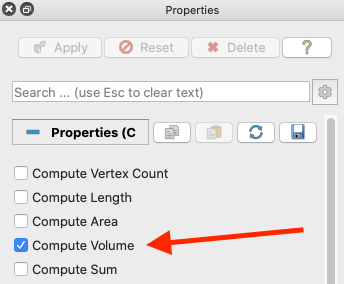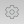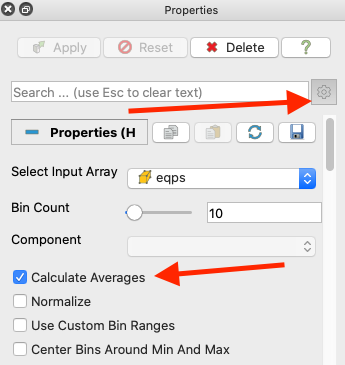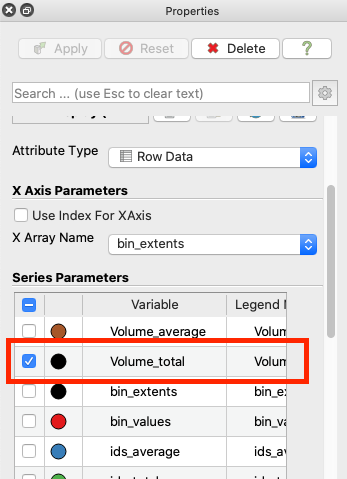# histogram: can we get an estimate volume per bin value?

Hi,
I would like to have a kind of histogram that display the distribution of data by the “percentage of the total volume” occupied by isovalues? For cell data, for instance, I would like to know in how much of the total volume the data is approximatively A.

Thanks for any suggestions
Best

PS: by the way, could someone explain what are bin_extends/bin_values?

I think the following sequence of steps will get what you want.

1. Calculate the volume of each cell. You can use the `Cell Size` filter to do that. It will create a cell field named `Volume` that represents the volume of each cell.The `Mesh Quality` filter can do something similar.

2. Add the `Histogram` filter. After selecting the input array and bin count, turn on the advanced options. Turn on the option for `Calculate Averages`. (It will calculate totals, too, which is what you want.)3. Once you apply the `Histogram` filter, you will see the bar chart now has several bars of different color for every bin. This is giving you, for each field, the average and summed value of data points that are in that bin. All you really want is the total volume for each bin. Scroll down the `Properties` panel until you get the table labeled `Series Parameters`. Turn off all series except the one named `Volume_total`.As for the question about `bin_extents` and `bin_values`, I forget the details, but they capture the bins of the histogram. `bin_extents` is capturing both the min and max value for each bin. In the histogram, you are not just finding for each bin i all field values that equal x_i. You are finding all values in the range from x_i to x_{i+1}. `bin_extents` is just capturing that range. If I recall, it is recording the center of the bin and some assumptions are made about the range by the bar chart view. The `bin_values` are just the computed value for each bin (i.e., the count).